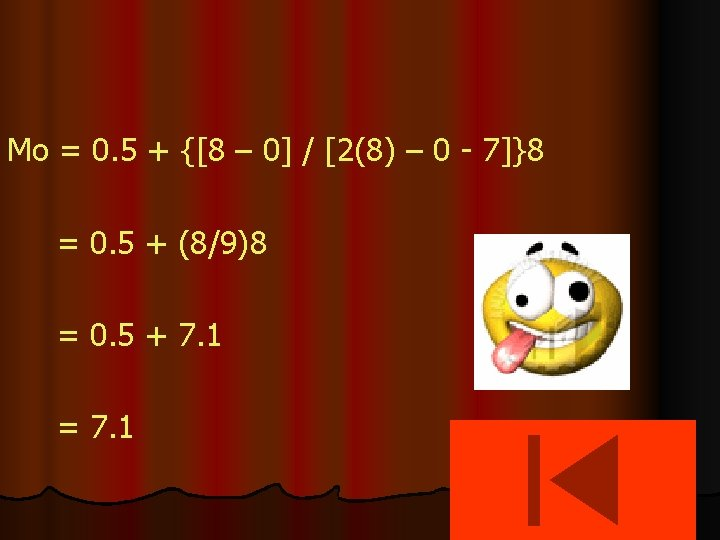# Measures of Central Tendency MODE Grouped Data Prepared

• Slides: 45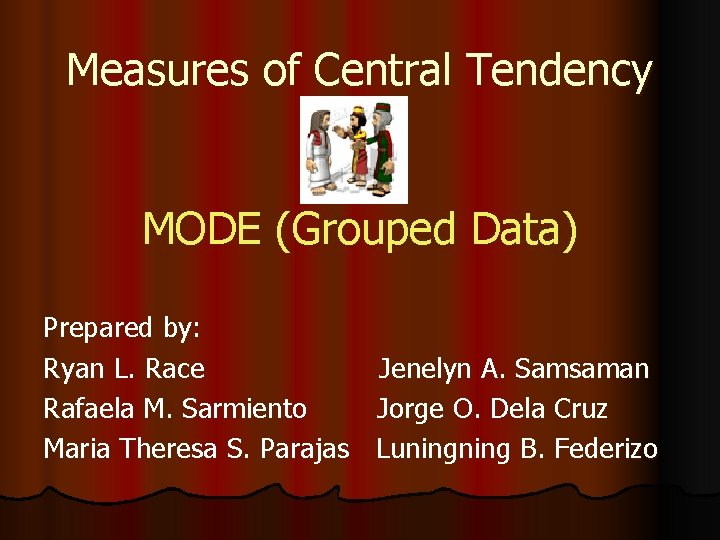Measures of Central Tendency MODE (Grouped Data) Prepared by: Ryan L. Race Jenelyn A. Samsaman Rafaela M. Sarmiento Jorge O. Dela Cruz Maria Theresa S. Parajas Luning B. FederizoPurpose/Rationale of this Module This module is designed to provide students with a step by step discussion on the computation of the mode for grouped data. It is to let the students discover for themselves how to compute for the mode (grouped data) through an easy visual presentation of the subject. Enjoy!What Will You Learn From This Module? After studying this module, you should be able to: 1. Compute the mode for grouped data. 2. Use mode for grouped data to analyze and interpret data to solve problems in daily life.Let’s See What You Already Know l Before you start studying this module, take the following test first to find out how prepared you are to solve for the mode of grouped data.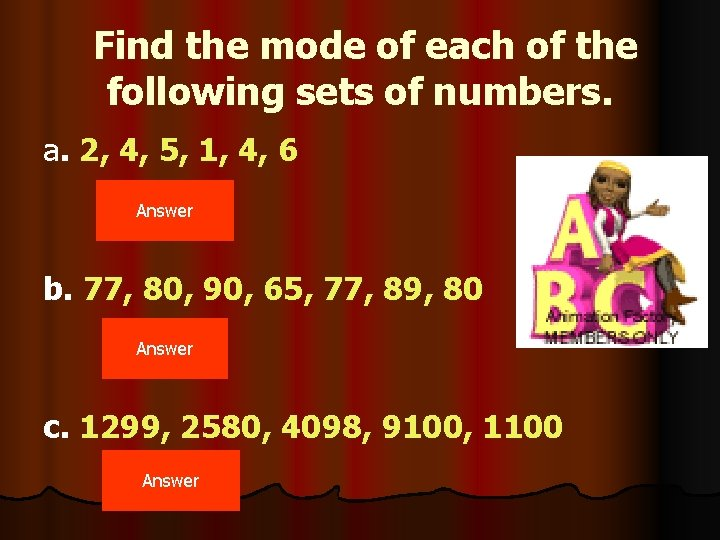Find the mode of each of the following sets of numbers. a. 2, 4, 5, 1, 4, 6 Answer b. 77, 80, 90, 65, 77, 89, 80 Answer c. 1299, 2580, 4098, 9100, 1100 AnswerAnswer: 4 Click me to answer letter b.Answer: 77 and 80 Click me to answer letter c.Answer: No Mode Go Back CONTINUEFor the next set of questions, refer to the given frequency distribution table of the grades of a group of students. CONTINUE1. What is the modal class? Grades 90 – 94 Number of students 2 85 – 89 5 80 – 84 8 75 – 79 12 70 – 74 3 ANSWER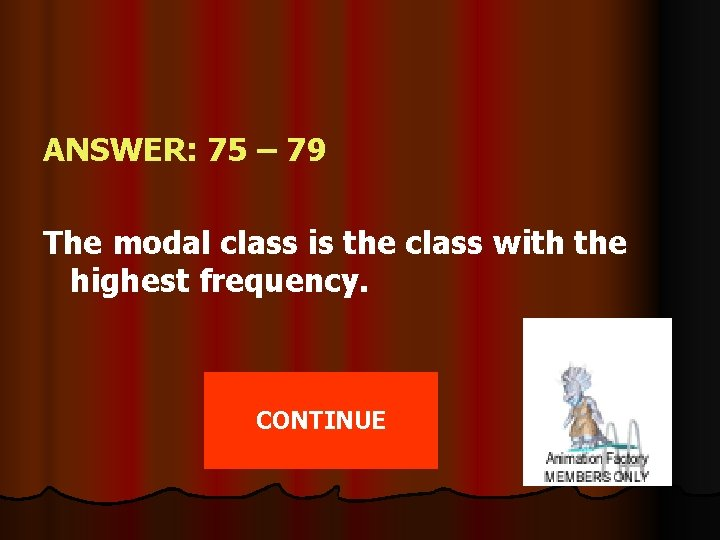ANSWER: 75 – 79 The modal class is the class with the highest frequency. CONTINUE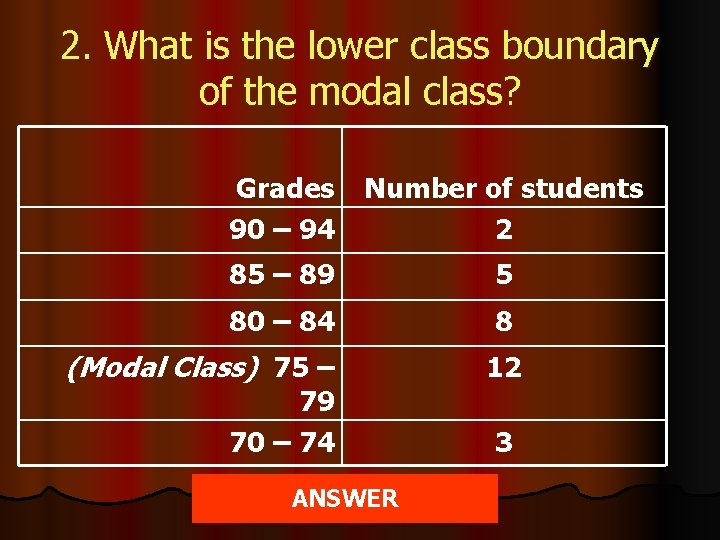2. What is the lower class boundary of the modal class? Grades 90 – 94 Number of students 2 85 – 89 5 80 – 84 8 (Modal Class) 75 – 79 70 – 74 ANSWER 12 3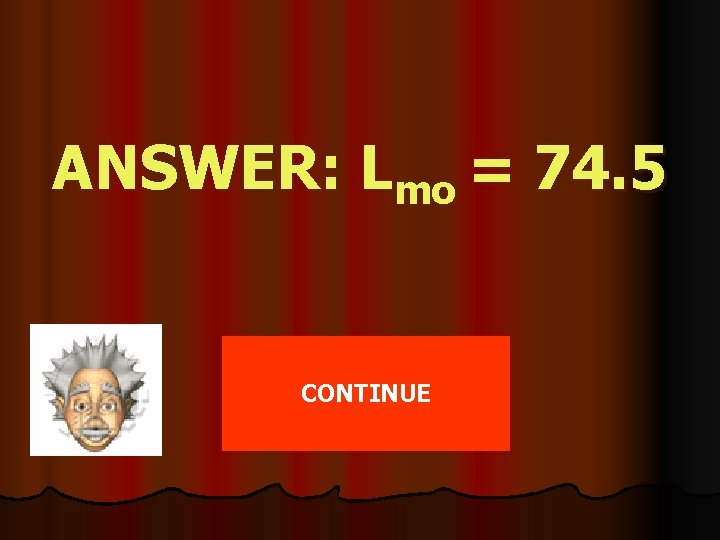ANSWER: Lmo = 74. 5 CONTINUE3. What is the frequency of the modal class? Grades 90 – 94 85 – 89 80 – 84 (Modal Class) 75 – 79 70 – 74 Number of students 2 5 8 12 3 ANSWER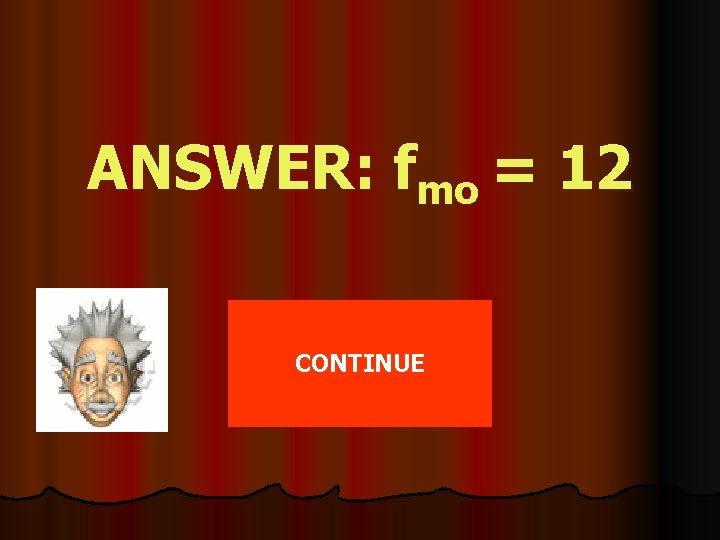ANSWER: fmo = 12 CONTINUE4. What is the frequency of the class preceding the modal class? Grades 90 – 94 85 – 89 80 – 84 (Modal Class) 75 – 79 70 – 74 Number of students 2 5 8 12 3 ANSWER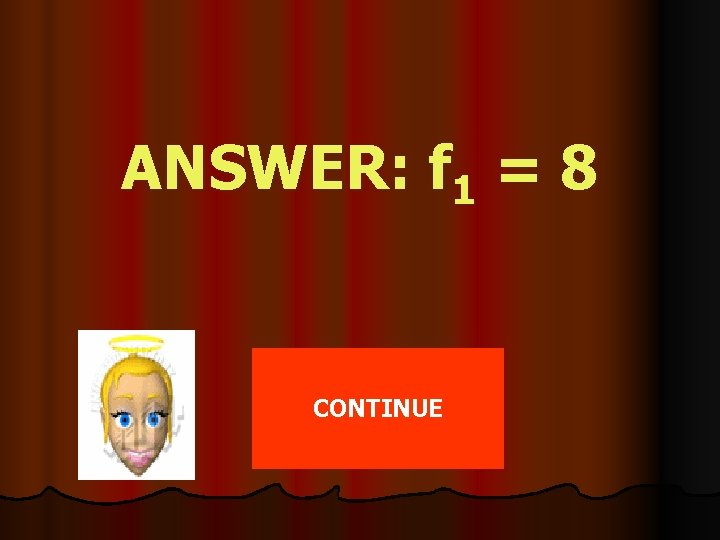ANSWER: f 1 = 8 CONTINUE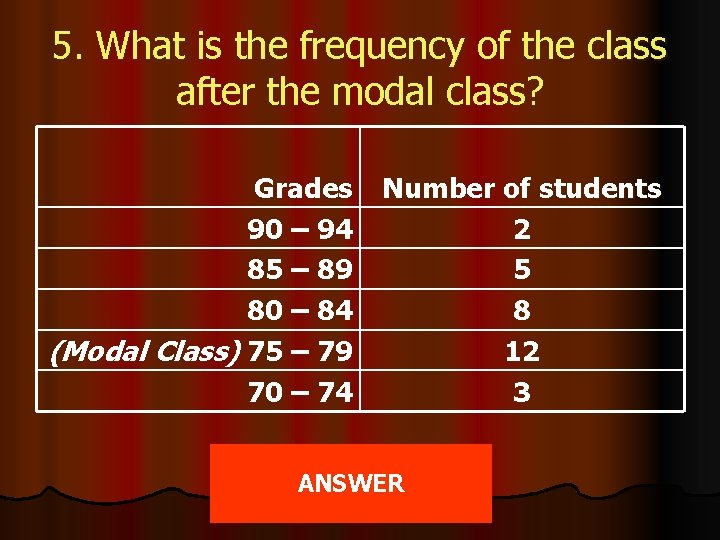5. What is the frequency of the class after the modal class? Grades 90 – 94 85 – 89 80 – 84 (Modal Class) 75 – 79 70 – 74 Number of students 2 5 8 12 3 ANSWER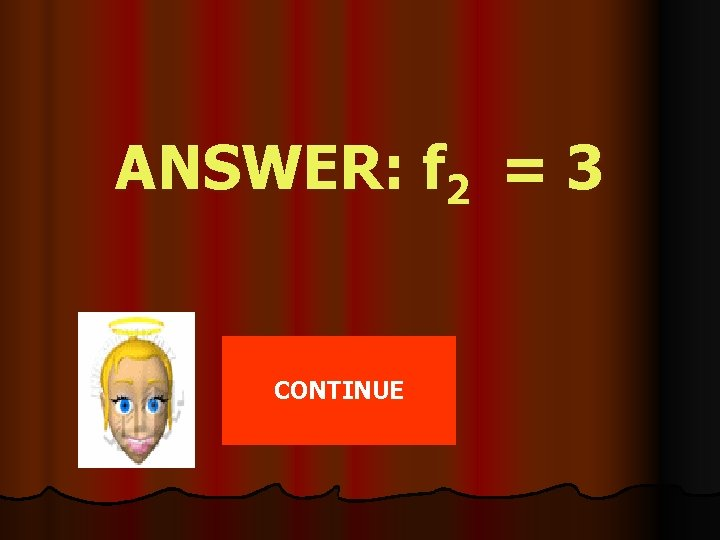ANSWER: f 2 = 3 CONTINUE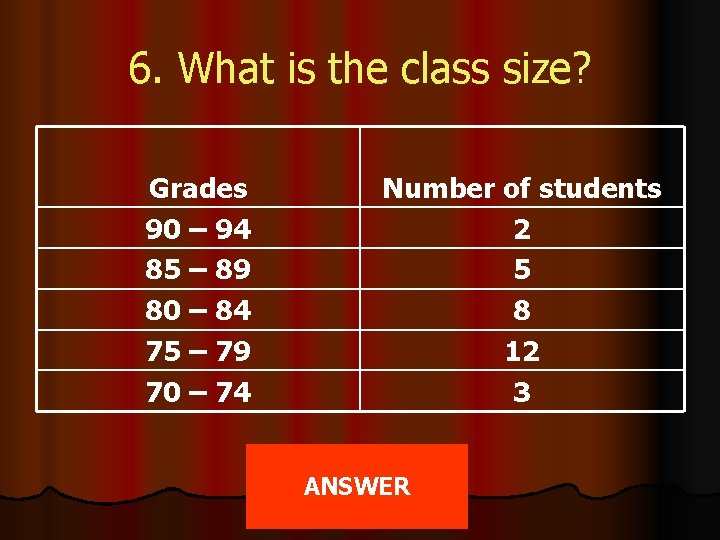6. What is the class size? Grades 90 – 94 85 – 89 80 – 84 75 – 79 70 – 74 Number of students 2 5 8 12 3 ANSWER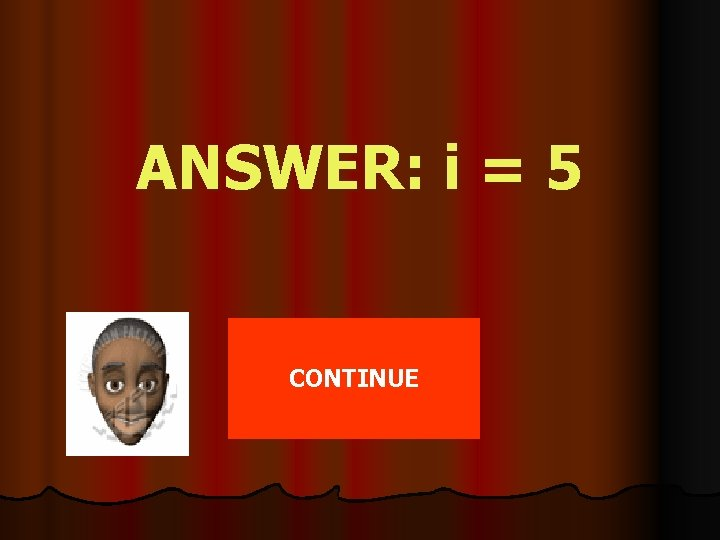ANSWER: i = 5 CONTINUE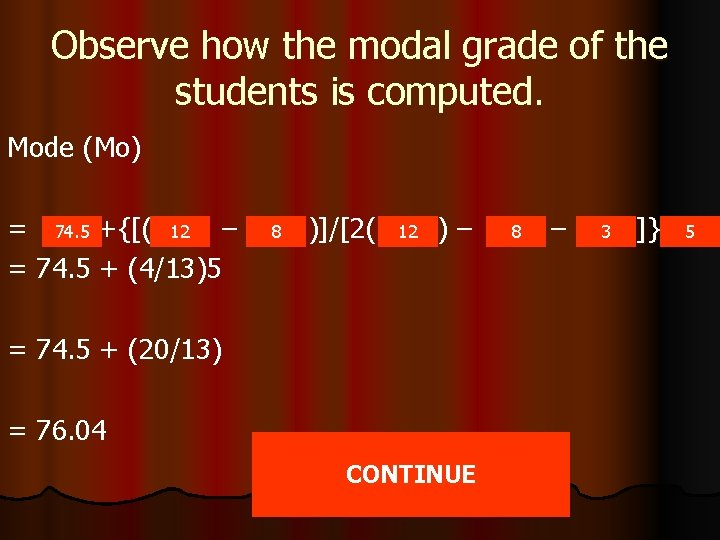Observe how the modal grade of the students is computed. Mode (Mo) = 74. 5 +{[( 12 – = 74. 5 + (4/13)5 8 )]/[2( 12 )– = 74. 5 + (20/13) = 76. 04 CONTINUE 8 – 3 ]} 574. 5 is the lower class boundary of the modal class (Lmo)12 is the frequency of the modal class (fmo)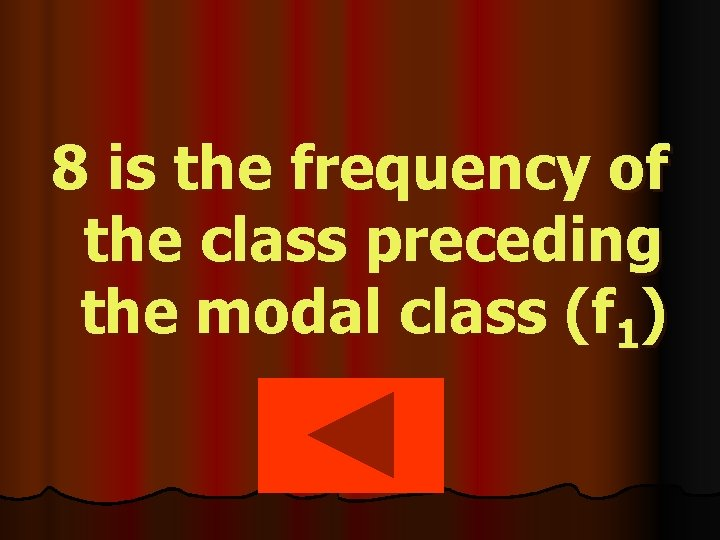8 is the frequency of the class preceding the modal class (f 1)3 is the frequency of the class after the modal class (f 2).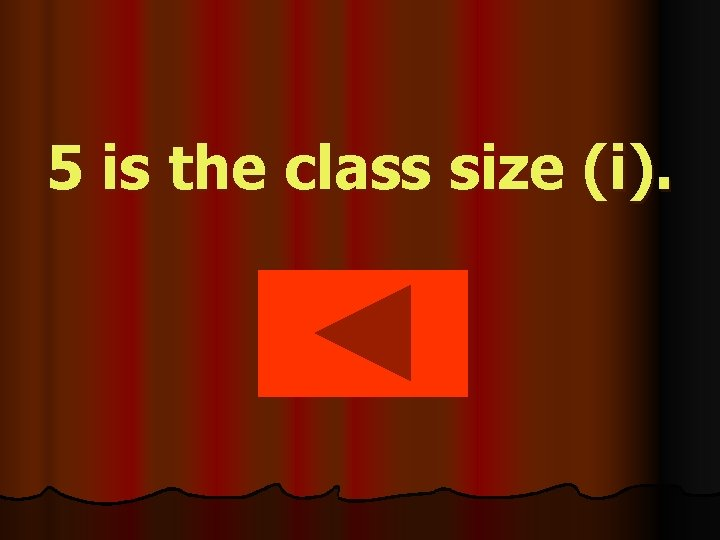5 is the class size (i).How did we solve for the modal grade of the students? Can you give the formula for the mode of grouped data? CONTINUETo solve for the mode of grouped data, use the formula Mo = Lmo+{( fmo – f 1 )/( 2 fmo – f 1 – f 2 )}i where, Lmo is the lower class boundary of the modal class, fmo is the frequency of the modal class, f 1 is the frequency of the class preceding the modal class, f 2 is the frequency of the class after the modal class, and i is the class size. CONTINUE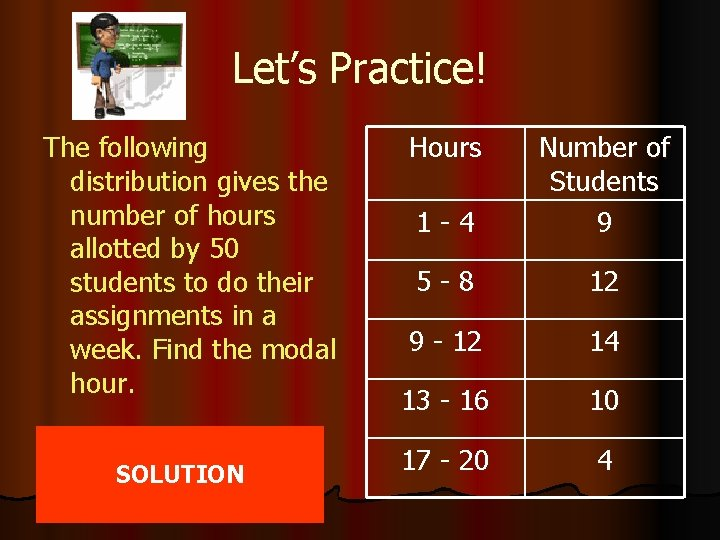Let’s Practice! The following distribution gives the number of hours allotted by 50 students to do their assignments in a week. Find the modal hour. SOLUTION Hours 1 -4 Number of Students 9 5 -8 12 9 - 12 14 13 - 16 10 17 - 20 4Modal Class : Lmo : f 1 : f 2 : i: Hours 1 -4 Number of Students 9 5 -8 12 9 - 12 14 13 - 16 10 17 - 20 4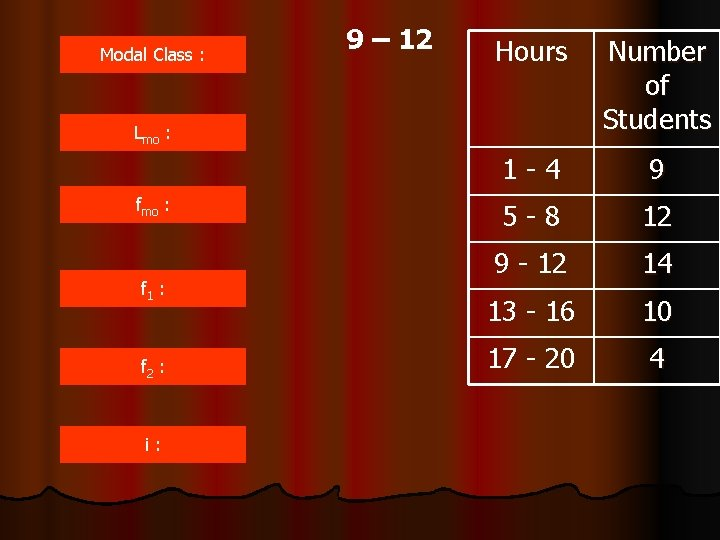Modal Class : 9 – 12 Hours Number of Students 1 -4 9 5 -8 12 9 - 12 14 13 - 16 10 17 - 20 4 Lmo : f 1 : f 2 : i: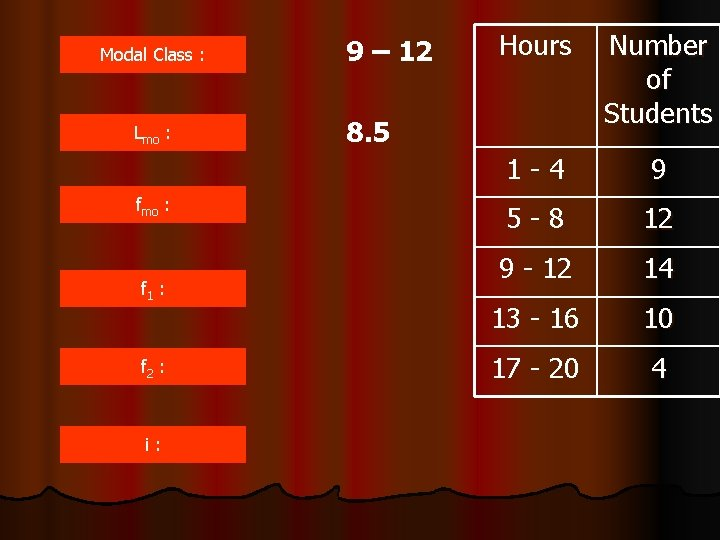Modal Class : Lmo : f 1 : f 2 : i: 9 – 12 Hours Number of Students 1 -4 9 5 -8 12 9 - 12 14 13 - 16 10 17 - 20 4 8. 5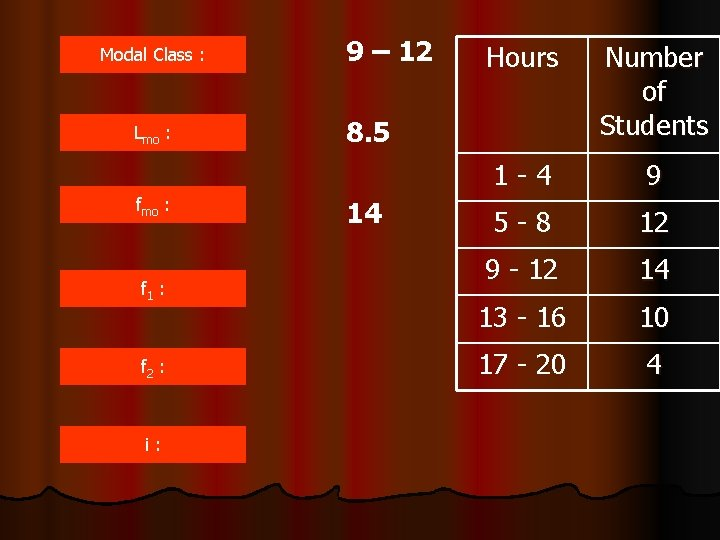Modal Class : Lmo : f 1 : f 2 : i: 9 – 12 Hours Number of Students 1 -4 9 5 -8 12 9 - 12 14 13 - 16 10 17 - 20 4 8. 5 14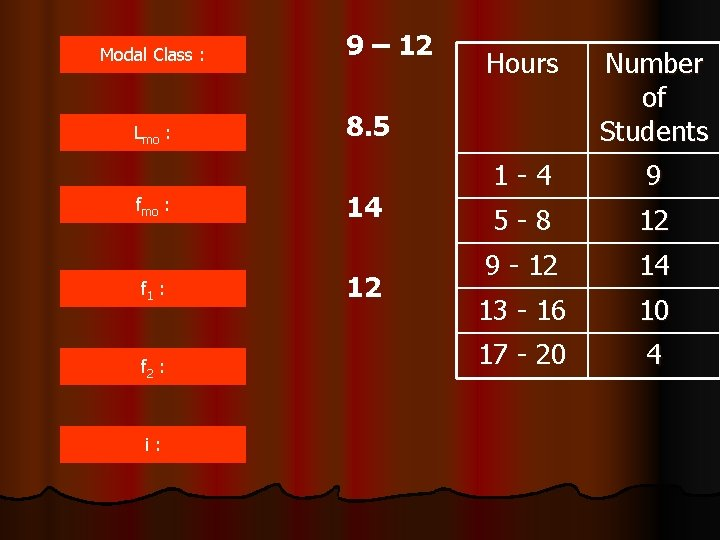Modal Class : Lmo : f 1 : f 2 : i: 9 – 12 Hours Number of Students 1 -4 9 5 -8 12 9 - 12 14 13 - 16 10 17 - 20 4 8. 5 14 12Modal Class : Lmo : fmo : 9 – 12 Number of Students 1 -4 9 5 -8 12 9 - 12 14 13 - 16 10 17 - 20 4 8. 5 14 f 1 : 12 f 2 : 10 i: Hours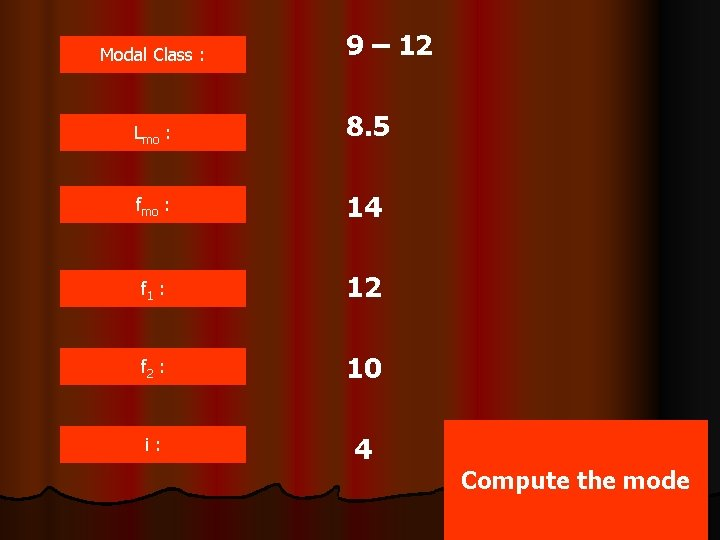Modal Class : 9 – 12 Lmo : 8. 5 fmo : 14 f 1 : 12 f 2 : 10 i: 4 Compute the mode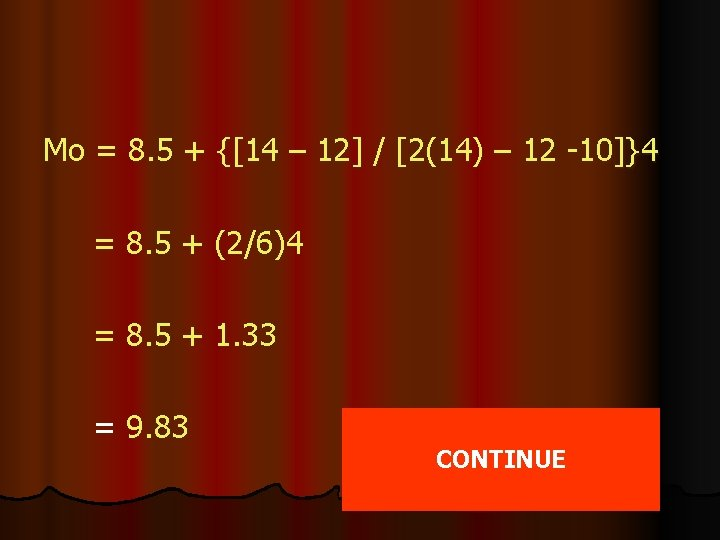Mo = 8. 5 + {[14 – 12] / [2(14) – 12 -10]}4 = 8. 5 + (2/6)4 = 8. 5 + 1. 33 = 9. 83 CONTINUEMore Practice? Find the mode using the frequency distribution of the heights of 40 students. SOLUTION SKIP Height(inches) No. of Students 52 – 54 2 55 – 57 4 58 – 60 3 61 - 63 8 64 – 66 10 67 – 69 9 70 - 72 4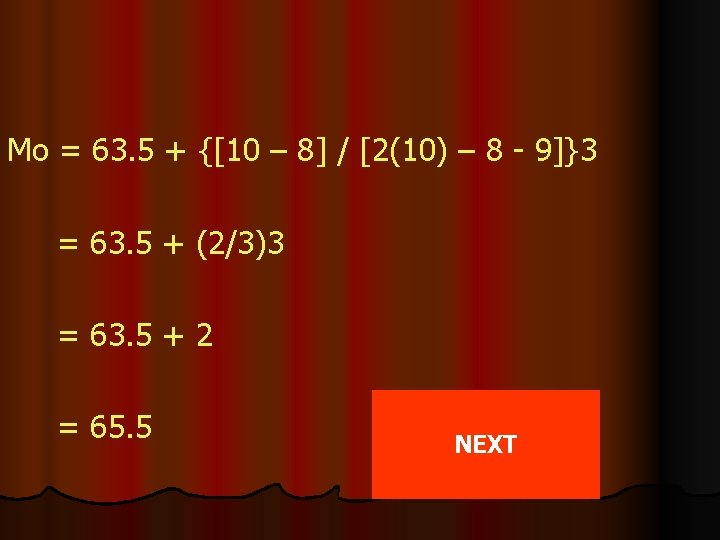Mo = 63. 5 + {[10 – 8] / [2(10) – 8 - 9]}3 = 63. 5 + (2/3)3 = 63. 5 + 2 = 65. 5 NEXT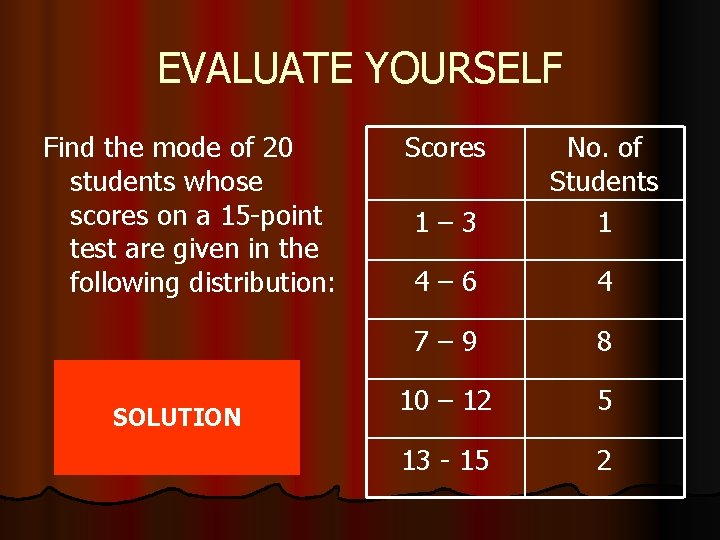EVALUATE YOURSELF Find the mode of 20 students whose scores on a 15 -point test are given in the following distribution: SOLUTION Scores 1– 3 No. of Students 1 4– 6 4 7– 9 8 10 – 12 5 13 - 15 2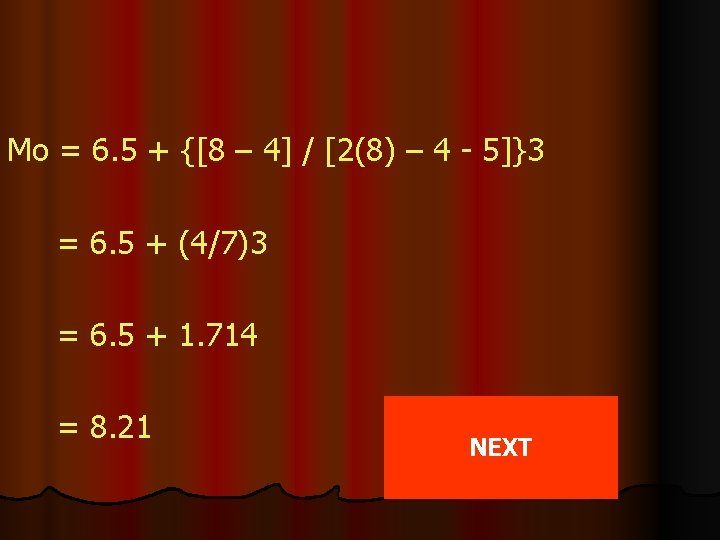Mo = 6. 5 + {[8 – 4] / [2(8) – 4 - 5]}3 = 6. 5 + (4/7)3 = 6. 5 + 1. 714 = 8. 21 NEXT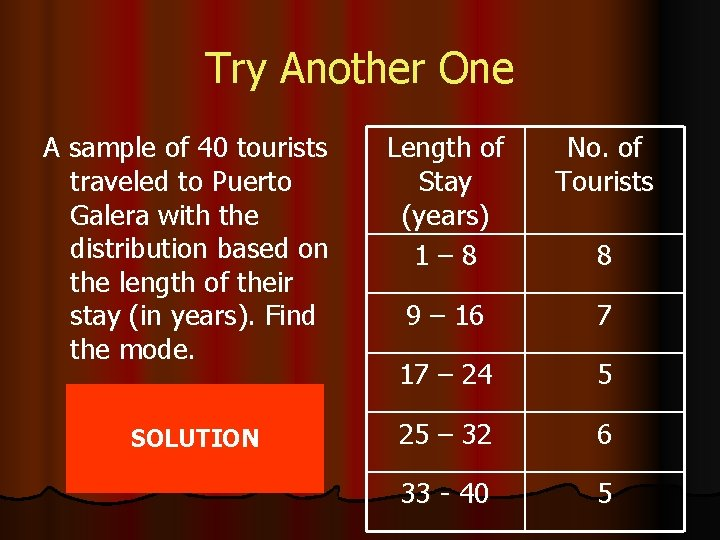Try Another One A sample of 40 tourists traveled to Puerto Galera with the distribution based on the length of their stay (in years). Find the mode. SOLUTION Length of Stay (years) 1– 8 No. of Tourists 9 – 16 7 17 – 24 5 25 – 32 6 33 - 40 5 8